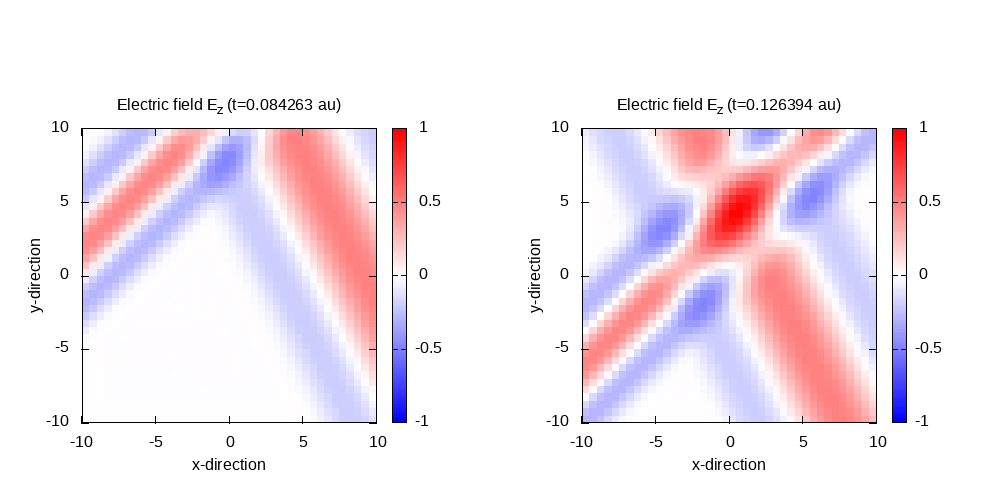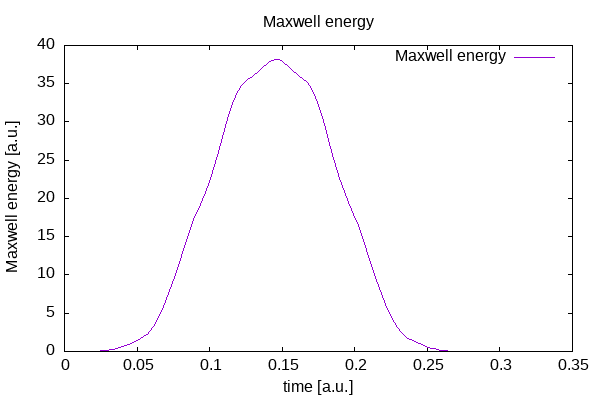# Interference of two cosinoidal plane waves

## Interference of two cosinoidal plane waves

Instead of only one plane wave, we simulate two different plane waves with different wave-vectors entering the simulation box, interfering and leaving the box again. In addition to the wave from the last tutorial, we add a second wave with different wave length, and entering the box at an angle, and shifted by 28 Bohr along the corresponding direction of propagation.

click for complete input

Both electric fields are polarized only in z-direction, and the magnetic field only in y-direction.

We can start from the last input file, and add the second wave, according to the following excerpt:


# ----- Maxwell field variables -------------------------------------------------------------------

# laser propagates in x direction

lambda1 = 10.0
omega1  = 2 * pi * c / lambda1
k1_x    = omega1 / c
E1_z    = 0.05
pw1     = 10.0
ps1_x   = - 25.0

# laser propagates in x-y direction

alpha = pi/4
lambda2 = 4.0
omega2  = 2 * pi * c / lambda2
k2_x    = omega2 / c * cos(alpha)
k2_y    = omega2 / c * sin(alpha)
E2_z    = 0.05
pw2     = 10.0
ps2_x   = - 28.0 * cos(alpha)
ps2_y   = - 28.0 * sin(alpha)

%MaxwellIncidentWaves
plane_wave_mx_function | 0 | 0 | E1_z | "plane_waves_function_1"
plane_wave_mx_function | 0 | 0 | E2_z | "plane_waves_function_2"
%

%MaxwellFunctions
"plane_waves_function_1" | mxf_cosinoidal_wave | k1_x | 0    | 0 | ps1_x | 0     | 0 | pw1
"plane_waves_function_2" | mxf_cosinoidal_wave | k2_x | k2_y | 0 | ps2_x | ps2_y | 0 | pw2
%


Contour plot of the electric field in z-direction after 50 time steps for t=0.11 and 100 time steps for t=0.21:Maxwell fields at the origin and Maxwell energy inside the free Maxwell propagation region of the simulation box: